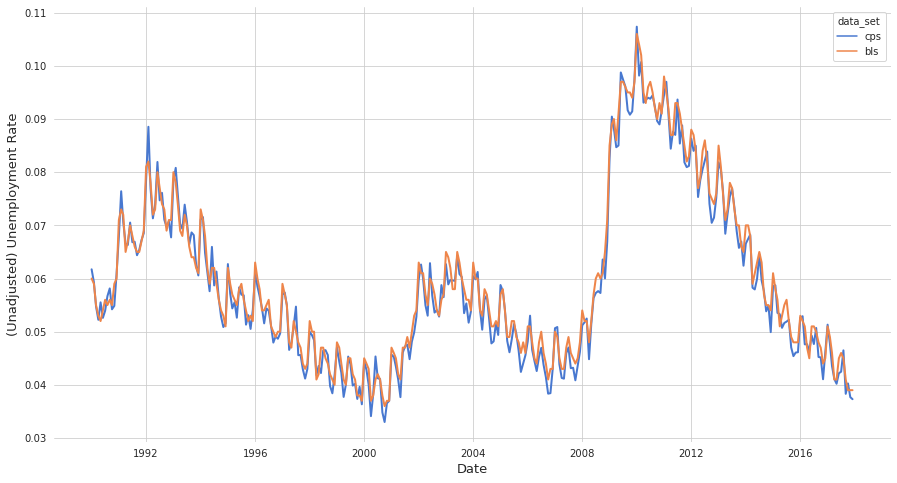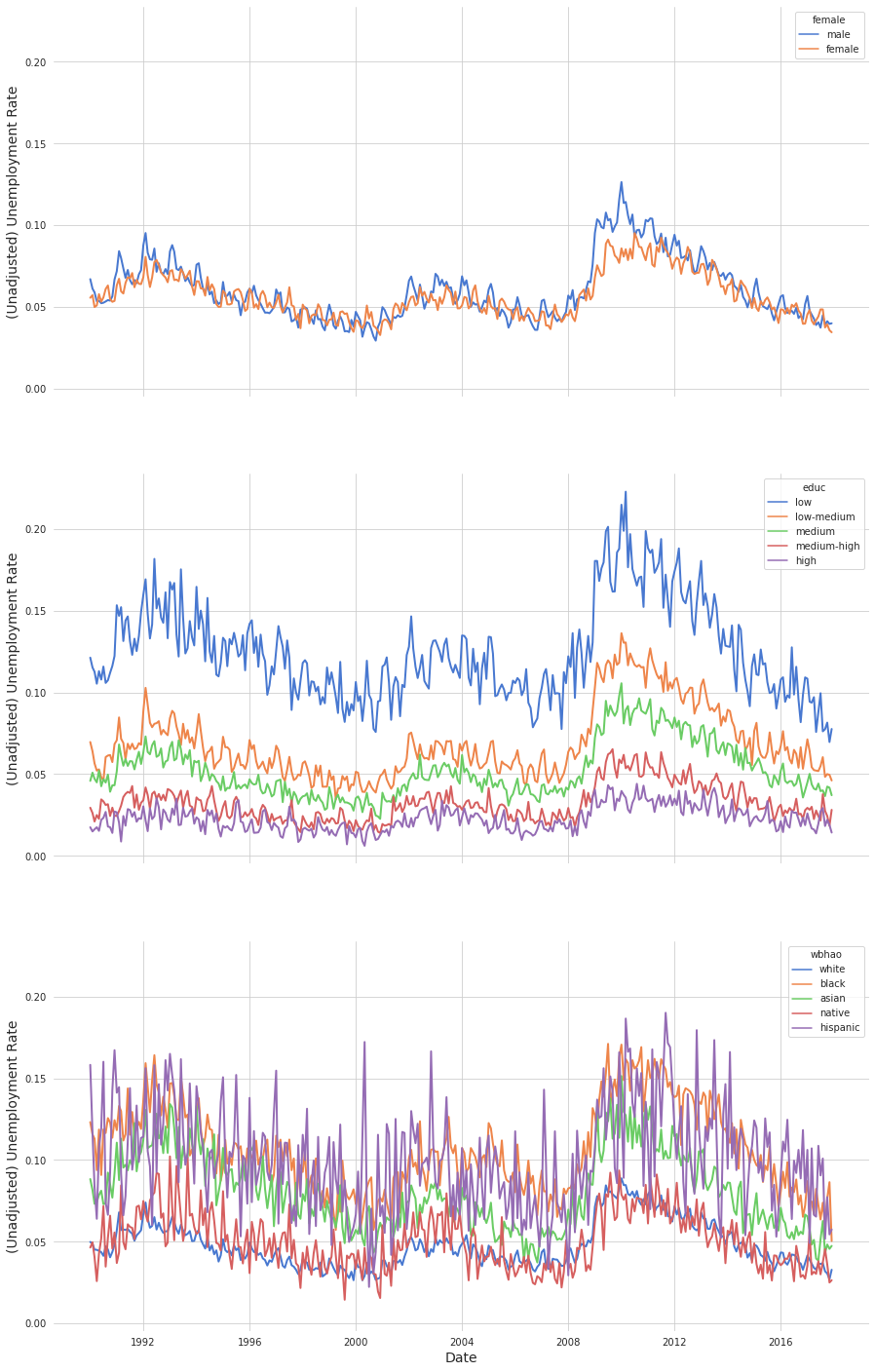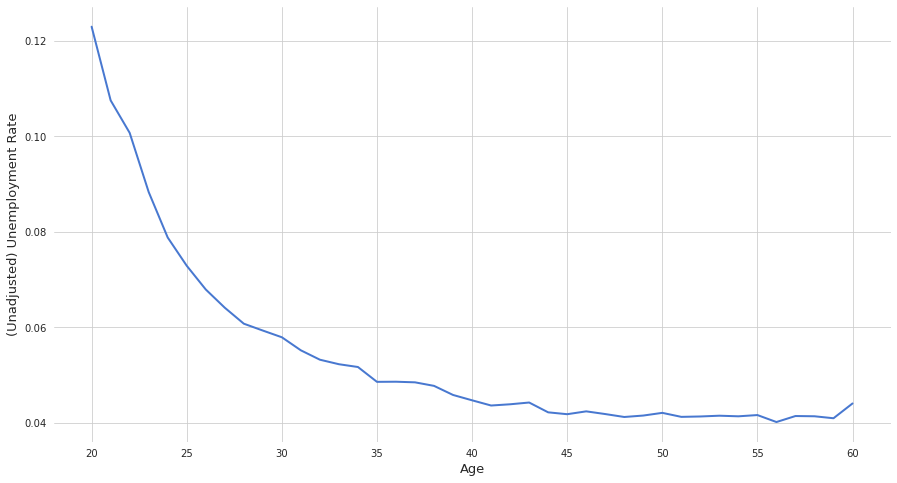### Notes on the Data:¶

The following notebook expects to find all data files in a folder with relative location data/data_ps3 but you may also simply pass the path of your data folder to the load_data function; this will work with unix and windows paths. Similarly, we also expect the xlsx file containing unemployment rates from the Bureau of Labor Statistics to be located at data/data_ps3/bls.xlsx -- this path can also be set in the load_data function.

If you want to download the data (at least the CPS data) on a unix machine without much hassle open your favorite terminal emulator and run the following commands line by line.

$for year in {1990..2017}; do$ wget "http://ceprdata.org/wp-content/cps/data/cepr_org_${year}.zip"$ done

$mkdir data_ps3$ mv *.zip data_ps3
$cd data_ps3$ unzip *.zip
\$ rm *.zip


### Preliminaries:¶

In :
import calendar

import numpy as np
import pandas as pd
import matplotlib.pyplot as plt
import seaborn as sns

from pathlib import Path
from functools import partial

sns.set_style("whitegrid")
sns.set_palette("muted")

In :
COLUMNS = [
"year",
"month",
"fnlwgt",
"empl",
"unem",
"female",
"wbhao",
"educ",
"age",
]

STR_MONTH_TO_INT = {(v, k) for k, v in enumerate(calendar.month_abbr)}


### Helper Functions:¶

In :
def load_data(cps_path="data/data_ps3", bls_path="data/data_ps3/bls.xlsx"):
"""Load CPS and BLS data.

Args:
cps_path (str or pathlib.Path): Path to folder containing the CPS data
for the years 1990 to 2017. Individual files in that folder have to
be named as e.g. 'cepr_org_2015.dta'.
bls_path (str or pathlib.Path): Path to the BLS data file.

Returns:
cps (pd.DataFrame): All data sets from 1990 to 2017 of the CPS data
concatenated over new time axis.
bls (pd.DataFrame): BLS data set.

"""
path = Path(cps_path)

merged_path = path / "cps_merged.pkl"
if merged_path.is_file():
cps = pd.read_pickle(merged_path)
else:
df_list = []
for year in range(1990, 2018):
df = pd.read_stata(
path / f"cepr_org_{year}.dta", convert_categoricals=False
)
df = df[COLUMNS]
df_list.append(df)
cps = pd.concat(df_list, axis=0)
cps[["empl", "unem", "educ"]] = cps[["empl", "unem", "educ"]].astype("Int64")
cps = convert_year_month_to_date(cps)

bls = pd.read_excel(bls_path, skiprows=11).rename(columns={"Year": "year"})
bls = bls.melt(id_vars="year", var_name="month", value_name="bls")
bls["month"] = bls["month"].replace(STR_MONTH_TO_INT)

return cps, bls

In :
def filter_data(df):
"""Filters data.

Drop observations with 'age' column below 16 and drop all observations
with NA entries in columns 'empl' or 'unem'.

"""
df = df.query("age >= 16")
df = df.dropna(subset=["empl", "unem"])
return df.copy()

In :
def convert_year_month_to_date(df):
"""Converts year and month columns to datetime index.

Args:
df (pd.DataFrame): Data set with at least columns 'year'
and 'month'.

Returns:
df (pd.DataFrame): The same data set but columns 'year'
and 'month' are replaced by a datetime object which
is set as the index.

"""
date = df["year"].astype(str) + "-" + df["month"].astype(str)
date = pd.to_datetime(date)
df["date"] = date
df = df.drop(["year", "month"], axis=1).set_index("date")
return df

In :
def compute_unemployment_rate_cps(df_filtered, covariate=None, replace_groupby=None):
"""Computes aggregate unemployment rate over indiviuals from filtered CPS data.

Args:
df_filtered (pd.DataFrame): Filtered CPS data set.

covariate (str or list of str): Column name or list of column names on which
the groupby operation should be performed. E.g. if 'covariate' == 'age',
then the groupby operation is performed along the columns ['year', 'month'
'age']. Defaults to None in which only ['year', 'month'] is used.

replace_groupby (str): Defaults to None in which case nothing happens. If not
set to None the entire groupby statement is replaced by 'replace_groupby'.
E.g. if 'replace_groupby' == ['age'], then 'df_filtred' is grouped only
by column 'age'.

Returns:
df (pd.DataFrame): The aggregated unemployment rate grouped by 'covariate'
or 'replace_groupby'.

"""
covariate = [] if covariate is None else covariate
covariate = covariate if isinstance(covariate, list) else [covariate]
keys = COLUMNS[:3]

def _ws(_data):
"""Weighted sum."""
weighted_sum = _data["fnlwgt"].dot(_data.drop("fnlwgt", axis=1))
return weighted_sum

gb_statement = (
["year", "month"] + covariate if replace_groupby is None else replace_groupby
)
gb = df_filtered.groupby(gb_statement)

summed = gb[["unem", "empl", "fnlwgt"]].apply(_ws)

summed.columns.name = None
summed["labor_force"] = summed["empl"] + summed["unem"]
summed["unem_rate"] = summed["unem"] / summed["labor_force"]

df = summed[["unem_rate"]].rename(columns={"unem_rate": "cps"}).reset_index()
return df

In :
def merge_cps_and_bls(cps, bls):
"""Merge CPS and BLS data set.

We assume that both input data sets were cleaned using function
convert_year_month_to_data and both columns 'unem_rate' are
measured on the same scale.

Args:
cps (pd.DataFrame): Clean CPS data set.
bls (pd.DataFrame): Clean BLS data set.

Returns:
df (pd.DatFrame): Both input data sets merged along 'date' axis.

"""
df = pd.concat((cps, bls), axis=1)
df = (
df.reset_index()
.melt(id_vars=["date"], var_name="data_set", value_name="unem_rate")
.set_index("date")
)
return df


### Comparison of CPS and BLS:¶

In :
cps_raw, bls_raw = load_data()

cps_filtered = filter_data(cps_raw)
cps = compute_unemployment_rate_cps(cps_filtered)
cps = convert_year_month_to_date(cps)

bls = convert_year_month_to_date(bls_raw)
bls /= 100

df = merge_cps_and_bls(cps, bls)

In :
fig, ax = plt.subplots(1)
fig.set_size_inches(15, 8)

sns.lineplot(
x="date",
y="unem_rate",
hue="data_set",
data=df,
ax=ax,
linewidth=2,
legend="brief",
)

ax.set(ylabel="(Unadjusted) Unemployment Rate", xlabel="Date")
ax.set_frame_on(False)
ax.xaxis.label.set_size(13)
ax.yaxis.label.set_size(13)
plt.show()## Unemployment Rate by Group:¶

In :
mapper = {
"wbhao": {1: "white", 2: "black", 3: "asian", 4: "native", 5: "hispanic",},
"female": {1: "female", 0: "male",},
"educ": {1: "low", 2: "low-medium", 3: "medium", 4: "medium-high", 5: "high",},
}

In :
covariates = ["female", "educ", "wbhao"]

dfs = {}
for covariate in covariates:
df_tmp = compute_unemployment_rate_cps(cps_filtered, covariate)
df_tmp = convert_year_month_to_date(df_tmp)
df_tmp = df_tmp.replace(mapper[covariate])
dfs[covariate] = df_tmp

In :
fig, axs = plt.subplots(3, sharex=True, sharey=True, figsize=(15, 25))

for i, (covariate, _df) in enumerate(dfs.items()):
ax = sns.lineplot(
x="date",
y="cps",
hue=covariate,
data=_df,
ax=axs[i],
linewidth=2,
legend="brief",
)

ax.set(ylabel="(Unadjusted) Unemployment Rate", frame_on=False)
ax.xaxis.label.set_size(14)
ax.yaxis.label.set_size(14)

plt.setp(axs[-1], xlabel="Date")
plt.show()### Unemployment Rate by Age:¶

In :
df_age = cps_filtered.query("20 <= age <= 60")

df_age = compute_unemployment_rate_cps(df_age, replace_groupby=["age"])

In :
fig, ax = plt.subplots(1)
fig.set_size_inches(15, 8)

sns.lineplot(
x="age", y="cps", data=df_age, ax=ax, linewidth=2,
)

ax.set(ylabel="(Unadjusted) Unemployment Rate", xlabel="Age")
ax.set_frame_on(False)
ax.xaxis.label.set_size(13)
ax.yaxis.label.set_size(13)
plt.show()## Average Unemployment for All Workers (older than 16):¶

In :
df.query("data_set == 'cps'").mean()

Out:
unem_rate    0.058877
dtype: float64